# Python Data Types (With Complete List)

Published on August 3, 2022By Pankaj
Developer and author at DigitalOcean.While we believe that this content benefits our community, we have not yet thoroughly reviewed it. If you have any suggestions for improvements, please let us know by clicking the “report an issue“ button at the bottom of the tutorial.

Python Data Types are used to define the type of a variable. In this article, we’ll list out all the data types and discussion the functionality of each. If you are starting out in Python, don’t forget to first visit the Python tutorial for beginners. And if you’ve already gone through the same, don’t forget to check out our previous tutorial on Python Comments and Statements.

## Python Data Types

There are different types of data types in Python. Some built-in Python data types are:

• Numeric data types: int, float, complex

• String data types: str

• Sequence types: list, tuple, range

• Binary types: bytes, bytearray, memoryview

• Mapping data type: dict

• Boolean type: bool

• Set data types: set, frozenset

### 1. Python Numeric Data Type

Python numeric data type is used to hold numeric values like;

1. int - holds signed integers of non-limited length.
2. long- holds long integers(exists in Python 2.x, deprecated in Python 3.x).
3. float- holds floating precision numbers and it’s accurate up to 15 decimal places.
4. complex- holds complex numbers.

In Python, we need not declare a datatype while declaring a variable like C or C++. We can simply just assign values in a variable. But if we want to see what type of numerical value is it holding right now, we can use type(), like this:

``````#create a variable with integer value.
a=100
print("The type of variable having value", a, " is ", type(a))

#create a variable with float value.
b=10.2345
print("The type of variable having value", b, " is ", type(b))

#create a variable with complex value.
c=100+3j
print("The type of variable having value", c, " is ", type(c))
``````

If you run the above code you will see output like the below image.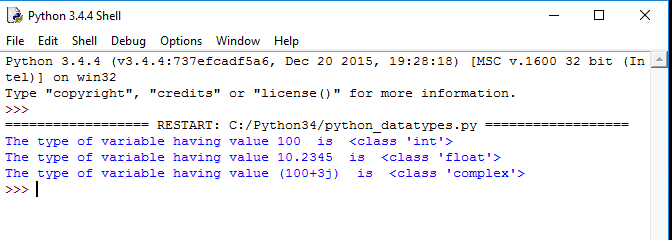### 2. Python String Data Type

The string is a sequence of characters. Python supports Unicode characters. Generally, strings are represented by either single or double-quotes.

``````a = "string in a double quote"
b= 'string in a single quote'
print(a)
print(b)

# using ',' to concatenate the two or several strings
print(a,"concatenated with",b)

#using '+' to concate the two or several strings
print(a+" concated with "+b)
``````

The above code produces output like the below picture-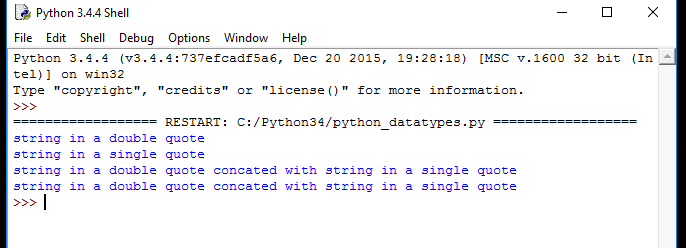### 3. Python List Data Type

The list is a versatile data type exclusive in Python. In a sense, it is the same as the array in C/C++. But the interesting thing about the list in Python is it can simultaneously hold different types of data. Formally list is an ordered sequence of some data written using square brackets([]) and commas(,).

``````#list of having only integers
a= [1,2,3,4,5,6]
print(a)

#list of having only strings
b=["hello","john","reese"]
print(b)

#list of having both integers and strings
c= ["hey","you",1,2,3,"go"]
print(c)

#index are 0 based. this will print a single character
print(c) #this will print "you" in list c
``````

The above code will produce output like this-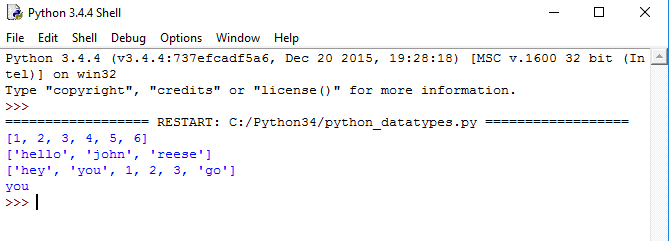### 4. Python Tuple

The tuple is another data type which is a sequence of data similar to a list. But it is immutable. That means data in a tuple is write-protected. Data in a tuple is written using parenthesis and commas.

``````#tuple having only integer type of data.
a=(1,2,3,4)
print(a) #prints the whole tuple

#tuple having multiple type of data.
b=("hello", 1,2,3,"go")
print(b) #prints the whole tuple

#index of tuples are also 0 based.

print(b) #this prints a single element in a tuple, in this case "go"
``````

The output of this above python data type tuple example code will be like the below image.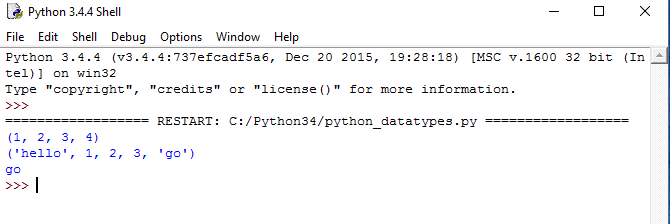### 5. Python Dictionary

Python Dictionary is an unordered sequence of data of key-value pair form. It is similar to the hash table type. Dictionaries are written within curly braces in the form `key:value`. It is very useful to retrieve data in an optimized way among a large amount of data.

``````#a sample dictionary variable

a = {1:"first name",2:"last name", "age":33}

#print value having key=1
print(a)
#print value having key=2
print(a)
#print value having key="age"
print(a["age"])
``````

If you run this python dictionary data type example code, the output will be like the below image.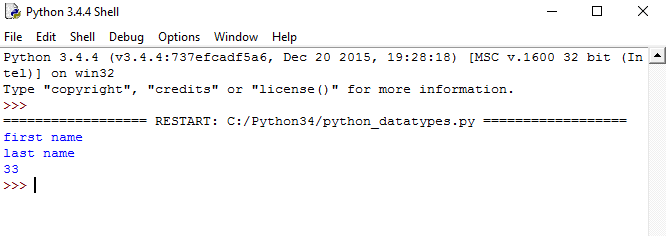So that’s all for today about Python data types. Don’t forget to run every piece of code on your own machine. Also, don’t just copy-paste. Try to write the lines of code on your own. #happy_coding :) Reference: Python Documentation for Data TypesPankaj

author

Developer and author at DigitalOcean.

#### Still looking for an answer?

@Shubham, This is not necessary. Double are required compulsory for text/strings whether you use them as Key or Value. Whenever you are using numeric as key or value, it is not mandatory that you have to use double quotes but if you are using string then you have to use it. For ex.: Case 1 : Numeric as Key without quotes and String as value a={1:“One”,2:“Two”,“Age”:35} >>> a ‘One’ Case 2: Numeric as Key in quotes and String as value >>> a={“1”:“One”,2:“Two”,“Age”:35} >>> a[“1”] ‘One’ Case 3: String as Key and Numeric as value >>> a={“One”:1,2:“Two”,“Age”:35} >>> a[“One”] 1 Hope it is clear. Thanks

- Nidhesh Tiwari

the code for dictionary data type is wrong …as the key is always surrounded by double quotes “key” correct code:- a = {“1”:“first name”,“2”:“last name”, “age”:33} #print value having key=1 print(a[“1”]) #print value having key=2 print(a[“2”]) #print value having key=“age” print(a[“age”])

- Shubham